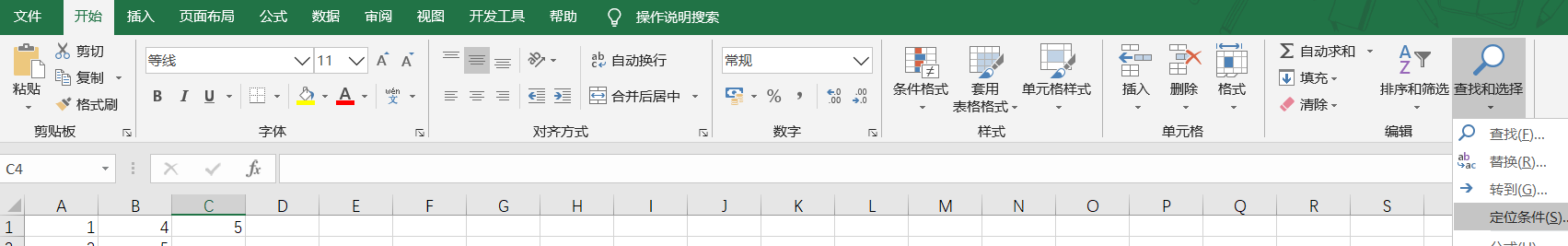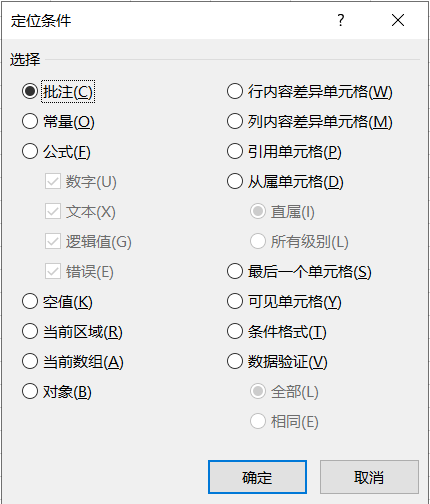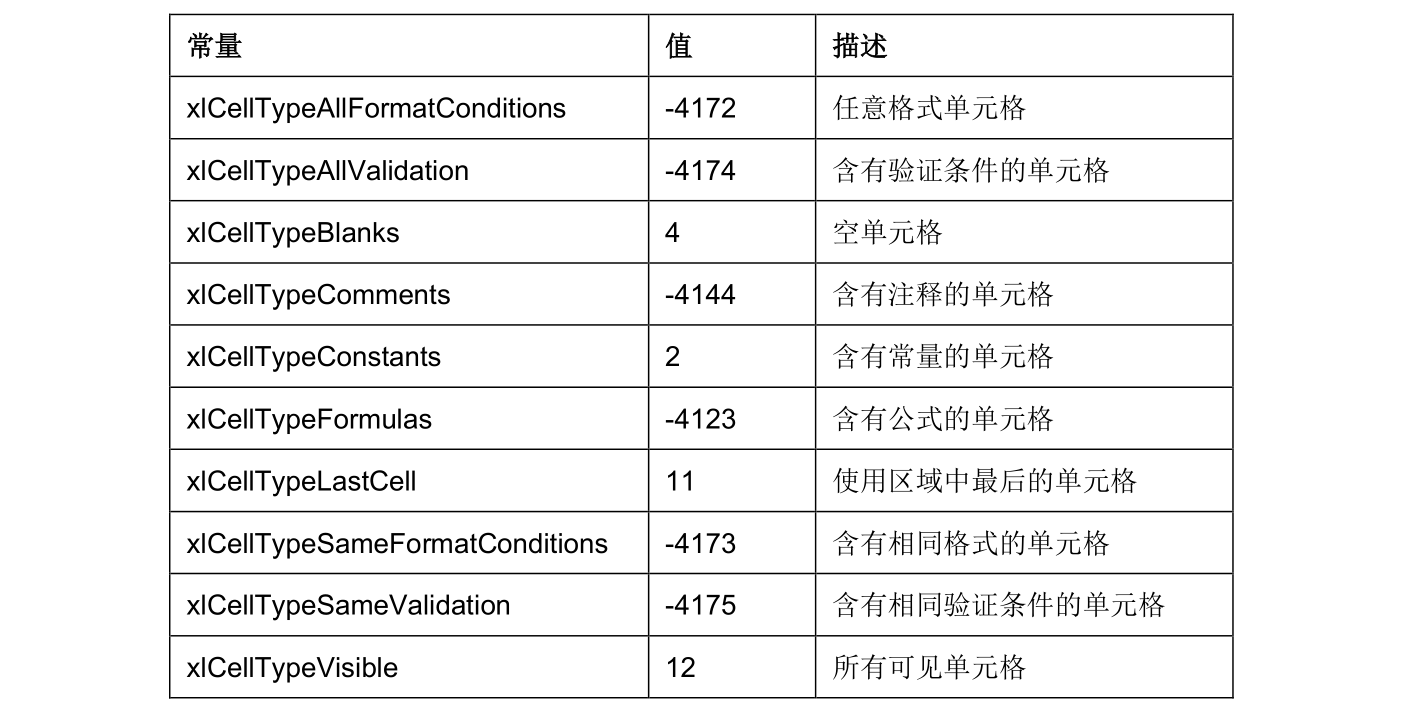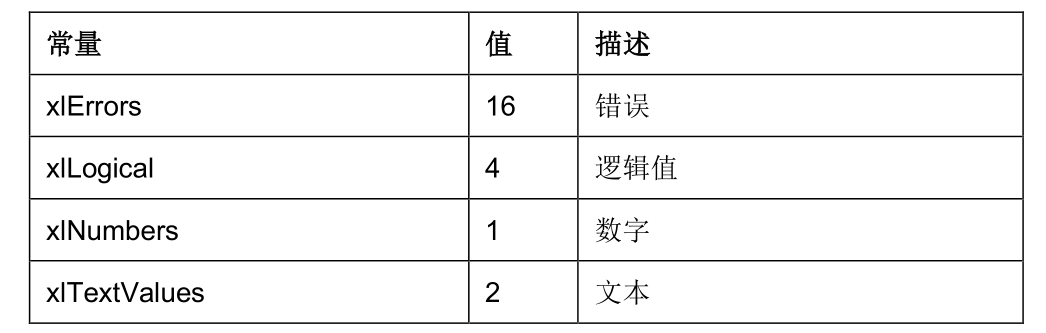• Sub 数组填充单元格() Dim aaa(3, 3) Dim temp(0, 2) aaa(0, 0) = "姓名" aaa(0, 1) = "llll" aaa(0, 2) = "jjjj" aaa(1, 0) = "性别" aaa(1, 1) = "男" aaa(1, 2) = "女" aaa(2, 0) = "序号" aaa(2, 1) =...
Sub 数组填充单元格()     Dim aaa(3, 3)     Dim temp(0, 2)      aaa(0, 0) = "姓名"      aaa(0, 1) = "llll"      aaa(0, 2) = "jjjj"      aaa(1, 0) = "性别"      aaa(1, 1) = "男"      aaa(1, 2) = "女"      aaa(2, 0) = "序号"      aaa(2, 1) = "1"      aaa(2, 2) = "2"      Range("a1").Resize(1, UBound(aaa, 2)) = aaa(1) '    temp(0) = aaa(0) '    aaa(0) = aaa(1) '    aaa(1) = temp(0) End Sub
展开全文excel
• 1.设置focus到第一个sheet的A1单元格。 Sub A1Select()  Dim shcl As Integer  For shcl = Sheets.Count To 1 Step -1  Worksheets(shcl).Activate  Range("A1").Select  ActiveWindow.S...
1.设置focus到第一个sheet的A1单元格。
Sub A1Select()
Dim shcl          As Integer
For shcl = Sheets.Count To 1 Step -1
Worksheets(shcl).Activate
Range("A1").Select
ActiveWindow.SmallScroll up:=10485
ActiveWindow.SmallScroll toleft:=100
Next shcl
Worksheets(1).Activate
ActiveWorkbook.Save
End Sub
2.文档保存前执行代码，打印出 test，该段代码必须位于ThisWorkBook（sheet）里面。
Private Sub Workbook_BeforeSave(ByVal SaveAsUI As Boolean, Cancel As Boolean)
MsgBox "test"
End Sub
3.文件打开时触发操作，打印出test。
Private Sub Workbook_Open()
Msg = "test"
MsgBox Msg, vbInformation
End Sub

展开全文• 不用多介绍…保存代码到VBA编辑器里, ALT+F8运行就好了… Public Sub InitCells() Dim iSheet As Long For iSheet = Sheets.Count To 1 Step -1 Sheets(iSheet).Activate 'ActiveWindow.Zoom = 10...
不用多介绍…保存代码到VBA编辑器里, ALT+F8运行就好了…
Public Sub InitCells()

Dim iSheet As Long

For iSheet = Sheets.Count To 1 Step -1

Sheets(iSheet).Activate
'ActiveWindow.Zoom = 100
ActiveWindow.ScrollRow = 1
ActiveWindow.ScrollColumn = 1
Sheets(iSheet).Range("A1").Activate

Next iSheet

End Sub

展开全文• ## VBA-定位单元格

千次阅读 2020-02-23 16:34:59
定位单元格 一、使用Excel定位对话框 在Excel自带功能中，即带有根据特殊格式定位的...二、使用VBA的SpecialCells 方法 该函数和下面Excel面板功能相通： 其使用格式为：expression.SpecialCells(Type, Value) ex...
定位单元格
一、使用Excel定位对话框
在Excel自带功能中，即带有根据特殊格式定位的功能：【开始】→【查找与选择】→【定位条件】。可以根据需要定位查找“标注”、“常量”、“公式”、“空值”、“可见单元格”等。二、使用VBA的SpecialCells 方法
该函数和下面Excel面板功能相通：
其使用格式为：expression.SpecialCells(Type, Value)
expression 是必需的，返回一个有效的对象。type也是必需的，在打出括号之后会有提示。参数 Value 是可选的，如果 Type 参数为 xlCellTypeConstants 或 xlCellTypeFormulas，此参数可用于确定结果中应包含哪几类单元格。将某几个值相加可使此方法返回多种类型的单元格。如果省略将选定所有常量或公式。 下表是type中可选的参数：下表是value可选的参数：Sub test()
Sheet1.Range("a1:c3").SpecialCells(xlCellTypeFormulas).Select '选择有公式的单元格
Sheet1.Range("a1:c3").SpecialCells(xlCellTypeFormulas, 1).Select '选择带有公式且为数字的单元格
Sheet1.Range("a1:c3").SpecialCells(xlCellTypeFormulas, 5).Select '选择带有公式且为数字和逻辑值的单元格
End Sub

展开全文• ## VBA锁定指定单元格

千次阅读 2019-05-14 17:41:00
If rs.RecordCount > 0 Then .Range("AF4").Value = pjno MsgBox "This project number does exists Please create a new one !", 16 .Range("A1:AH56").L...
• 在Visual Basic中，Range对象既可表示单个单元格，也可表示单元格区域。  引用 含义 Range("A1") 单元格A1 Range("A1:B5")   从单元格A1单元格B5的区域 Range("C5:D9,G9:H16") 多块选定区域 Range...
• 很多同学想学习VBA，却没有勇气，下面是我VBA入门直播课程中的知识点，参加了培训的同学请自己对照下是否掌握了。其它有基础知识的同学也可以自检下。(报名培训班请加微信：527240310)第一讲：...
•EXCEL 设置 格式
• 获取代码如下 ... [a6] = Target.Address(0, 0) '获取选中单元格名称（字符名称 例如 C5 ) [a7] = ActiveCell.Cells.Row '获取选中单元格的行编号 数值 [a8] = ActiveCell.Cells.Column '获取选中单元.
• Range.Merge 方法：由指定的Range对象创建合并单元格 （一）对选择的区域进行合并 Sub 合并单元格() Selection.Merge '对选中的单元格区域执行此段代码，进行合并，仅保留左上角值 End Sub ******************...
• '1 表示一个单元格(a1) Sub s() Range("a1").Select Cells(1, 1).Select Range("A" & 1).Select Cells(1, "A").Select Cells(1).Select [a1].Select End Sub '2 表示相邻单元格区域 Sub d() '
• 下例向第一张工作表上的单元格 A1 添加双边框。 Worksheets(1).Range("A1").Borders.LineStyle = xlDouble 使用 Borders ( index )（其中 index 指定边框）可返回单个 Border 对象。下例设置单元格区域 ...
• Value是单元格的数字内容 Text是文本内容 Formula是最原本的,输入内容 Sub 宏3() '测试，就不去定义变量类型了 a = Range("b1").Value b = Range("b1").Text c = Range("b1").Formula End Sub ...
• 要求：一个Excel文件中，有多个sheet，每个sheet中都有特定范围的单元格需要填充颜色，手动填充太麻烦，下面是VBA实现的自动填色 1 Sub incolor() 2 3 Dim i, j, sheetnum, rownum 4 5 '获取当前文档中的...
• VBA编程经常要读取、写入单元格内容，所以总结这么一篇笔记，我只是把最常用到的功能列举出来。具体用法有疑惑可以百度。 ThisWorkbook：当前工作簿，这个跟VBA代码编写位置有关系，如下图所示： ActiveWorkbook：...
• vba设置单元格字体，以下是设置给定单元格内字体的加粗，单元格填充灰色等，参数是Range对象 Sub font_sty(Rng As Range) Rng.Select Selection.Font.Bold = True With Selection.Interior .Pattern = xlSolid...
• 禁用单元格拖拽功能 在工作表中可以拖放单元格右下角的小十字对单元格内容进行复制等操作，如果不希望用户进行此操作可以禁用单元格拖放功能。... If Not Application.Intersect(Target, Range("A1:A15")) Is Nothi...
•  f = Dir(file(1) & "*.xlsx") '通配符*.*表示所有文件，*.xlsx Excel文件  Do Until f = ""  Cells(2, x).Hyperlinks.Add Anchor:=Cells(2, x), Address:=file(i) & f, TextToDisplay:=f  Temp = lj & "\...
• VBA-Excel中单元格的引用方法 使用VBA对Excel中的单元格单元格区域进行选择有很多种方法，可以归结为两大类： 使用行列数进行选择 根据已经选择的单元格(区域)进行再选择 1、使用range属性 VBA中可以使用range...
•  Set rg = .Range("A1:A" & iNum + 100)  For Each cell In rg  cell.Locked = True  Next  ActiveSheet.Protect DrawingObjects:=True, Contents:=True, Scenarios:=True _  , AllowInsertingRows:...
• Range(“a1:a10”).Select '表示选中单元格A1:A10的区域 Range(“a2”).Value = 1 '表示单元格赋值为1，Range的默认属性就是Value Range(“a1”).Offset(2, 3).Select '以单元格a1为基准，下移2行，右移3列，...excel
• 如何选择当前工作表中的单元格？ 例如，可以使用下面的代码选择当前工作表中的单元格D5： ActiveSheet.Cells(5, 4).Select 或：ActiveSheet.Range("D5").Select 如何选择同一工作簿中其它工作表上的单元格？ ...
• VBA操作Excel之获取单元格区域一、VBA通过索引引用工作表二、VBA获取单元格区域三、VBA调用方法参数传值参考文档 一、VBA通过索引引用工作表 VBA打开工作簿后，通常一个工作簿有若干个工作表Sheet，访问其中一个工作...索引 excel
• 目录 单元格对象的Value属性 单元格对象的Text属性 ...如获取A1单元格的值，可以用： Range("A1").Value Value属性为单元格对象的默认属性，当需要获取某个对象的默认属性时，可以直接用对象表示。 非特...
• ## 10, excel vba 修改单元格的颜色

万次阅读 多人点赞 2018-03-07 19:07:19
看数据总是很累的,一堆密密麻麻的.哪个数据才是我想要的呢? 比如一列里有各种各样的数据,我想知道哪些单元格的数据大于11的?...在vba中一种颜色都对应着一个数字或者说序列吧. 比如红色. 它对应的序列...excel
• 你的VBA过程可能需要查明某个具体单元格的格式。我们可以使用NumberFormat属性来找回单元格格式： ?Range("A1").NumberFormat 在立即窗口输入上面的问题后，Excel显示“General” Range("A1").NumberFormat =...excel 程序设计
• VBA 中一般使用 Select 方法选定单元格单元格区域，格式为：.select。但是需要注意的是在使用该函数之前保证单元格所在工作表为活动工作表。 Sub test1() Range("a1:d4").Select Sheet2.Activate '激活工作表...
• ## VBA 设置单元格格式

千次阅读 2019-05-21 15:37:18
庆年工坊 知道合伙人互联网行家推荐于2017-10-02 1 2 3 4 5 ... [a1].NumberFormatLocal="@"'设置A1单元格为文本格式 [b1].NumberFormatLocal="yyyy/m/d"'设置B1单...
• '单元格合并 Sub h1() Range("g1:h3").Merge ' End Sub '合并区域的返回信息 Sub h2() Range("e1") = Range("b3").MergeArea.Address '返回单元格所在的合并单元格......